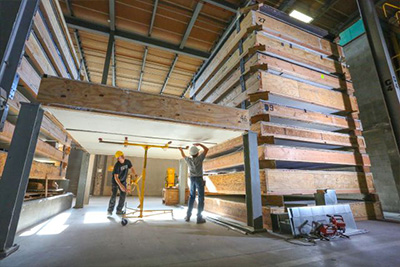# When Is A Decibel Not A Decibel?

September 6, 2021   -

In the Acoustics Lab of NGC Testing Services, we are concerned primarily with the measurement of Sound Pressure Level and Sound Power Level. "Sound Power" and "Sound Pressure" are two distinct and commonly confused characteristics of sound. Both share the same unit of measure, the decibel (dB), and the term "Sound Level" is commonly substituted for each.

So what is the difference between a Sound Pressure Decibel and a Sound Power Decibel?

## What is a decibel?A decibel is defined as "a unit of measurement used to express the ratio of one value of a physical property to another on a logarithmic scale. It expresses the ratio of a value to a reference value." The decibel is used for a wide variety of measurements in science and engineering, most prominently in acoustics, electronics, and control theory. In electronics, the gains of amplifiers, attenuation of signals, and signal-to-noise ratios are often expressed in decibels. A decibel is a unit-less quantity since it is a ratio.

## Sound Power Decibel

Sound Power Decibel Level is the absolute measurement of the total acoustic energy or wattage that a sound source is emitting. Since it is the measure of the total acoustic energy being radiated, it is not affected by the acoustic environment in which it is located. It is an absolute.

## Sound Pressure Decibel

On the other hand, Sound Pressure Decibel Level is a pressure disturbance in the atmosphere whose intensity is influenced not only by the strength of the source but also by the surroundings and the distance from the source to the receiver. It is simply the Sound Pressure Level measured of a Sound Power Source at a particular location (orientation and distance) in a particular acoustic environment.

If you know the sound power that is emitted by a sound source, it is possible to estimate the Sound Pressure Level that would be measured at a certain distance from that source in a certain acoustic environment (room size, room dimensions, and room absorption). This is an important tool for the prediction of noise levels when a sound source is introduced to an already noisy environment.